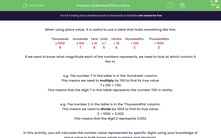# Understand Place Value

In this worksheet, students will calculate the number value represented by specific digits, using knowledge of place value in both larger whole numbers and decimals.Key stage:  KS 4

Year:  GCSE

GCSE Subjects:   Maths

GCSE Boards:   Pearson Edexcel, OCR, Eduqas, AQA,

Curriculum topic:   Number, Number Operations and Integers

Curriculum subtopic:   Structure and Calculation Whole Number Theory

Popular topics:   Place Value worksheets

Difficulty level:#### Worksheet Overview

When using place value, it is useful to use a table that looks something like this:

 Thousands Hundreds Tens Units . Tenths Hundredths Thousandths x 1000 x 100 x 10 x 1 . ÷ 10 ÷ 100 ÷ 1000 8 7 6 5 . 4 3 2

If we want to know what magnitude each of the numbers represents, we need to look at which column it lies in.

e.g. The number 7 in the table is in the 'Hundreds' column.

This means we need to multiply by 100 to find its true value.

7 x 100 = 700

This means that the digit 7 in this table represents the number 700 in reality.

e.g. The number 2 in the table is in the 'Thousandths' column.

This means we need to divide by 1000 to find its true value.

2 ÷ 1000 = 0.002

This means that the digit 2 represents 0.002.

In this activity, you will calculate the number value represented by specific digits using your knowledge of place value in both larger whole numbers and decimals.

Let's get going!

### What is EdPlace?

We're your National Curriculum aligned online education content provider helping each child succeed in English, maths and science from year 1 to GCSE. With an EdPlace account you’ll be able to track and measure progress, helping each child achieve their best. We build confidence and attainment by personalising each child’s learning at a level that suits them.

Get started